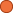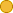## ← Fractals08 02EmpiricalScaling01

• 1 Follower
• 133 Lines

### Get Embed Code x Embed video Use the following code to embed this video. See our usage guide for more details on embedding. Paste this in your document somewhere (closest to the closing body tag is preferable): ```<script type="text/javascript" src='https://amara.org/embedder-iframe'></script> ``` Paste this inside your HTML body, where you want to include the widget: ```<div class="amara-embed" data-url="http://www.youtube.com/watch?v=NKqh-2FlC7U" data-team="complexity-explorer"></div> ``` 4 Languages

•English [en] original
•Arabic [ar]
•Spanish [es] incomplete
•Portuguese, Brazilian [pt-br] incomplete

Showing Revision 7 created 03/21/2017 by Cigdem Yalcin.

1. The starting point for urban scaling is similar
2. to the starting point for metabolic scaling.
4. of something depend on their size.
5. For urban scaling the size
6. it will be interested in is their population
7. and we’ll look at different properties.
8. wages, GDP, length of roads,
9. amount of electricity used and so on.
10. So in this video I want to just take an empirical look at this
12. So I’ll do so by showing you several plots
13. And as usual I’ll put the reference with the plots down here.
14. So here is the first one.
15. This is looking at population, down here.
16. And this is total wages and this is for US cities
17. And in this context the city is taken
18. to be a metropolitan statistical area,
19. So it might not be exactly the same as a formal city boundary,
20. often might includes suburbs.
21. If you have two cities are right next to each other
22. they will be considered the part of the same metropolitan area.
23. So any event, here is the data,
24. I think we got around 300 data points here
25. And this is on a log-log plot
26. And we can definitely see a linear trend.
27. We can calculate the slope,
28. which we know as the exponent in a power law
29. And that gives us a Beta at 1.12
30. So note here, this is larger than 1,
31. So what this means is
32. if we have a small city
33. and then we do compare to that city that twice as large
34. we might ask, well, how would the wages,
35. the total wages, total amount of money
36. made in both of those cities compared.

37. And you might think well,
38. the city that has twice as many people
39. should have wages that are twice as much.
40. What this says, it’s actually more than that,
41. it's faster than linear, super linear.
42. So that if you double the population
43. on average according to this trend,
44. you would more than double the total wages
45. It would go by to do though the 1.12 not to do the 1
46. Alright so, that’s sort of interesting I think
47. and others have thought.
48. Because we might expect that it would be linear
49. doubling population with double wages,
50. but that’s definitely now what we see
51. of course that can’t help but notice that
52. there is an awful a lot fuzz around this line.
53. so there’s a very clear trend
54. that’s pretty hard to deny
55. but it’s not an exact relationship like a physical law might be
56. there is even more scattered I think
57. than for most of the metabolic scaling plots.
58. So there’s a lot of variation among cities as well.
59. And there is a clear trend.
60. And as we talked about in metabolic scaling
61. the trend can be interesting
62. and the deviations from the trend can be interesting
63. and those two statements don’t need
64. to be in competition to each other.
65. Both can be interesting.
66. In this case I think both are interesting.
67. Ok, let’s look at a few other results.
68. And there are lots of lots of data sets like this
69. But I’ll show you a few more.
70. Alright, again we have population on the horizontal axis.
71. A log-log scale
72. This is log not a wages but it’s GDP, gross domestic product
73. And these are for Chinese cities
74. so this is measured in million Yuan.
75. And again we can see there is a very clear trend.
76. It’s certainly not a flat line. Beta is 1.12
77. But for this data set there is even more
79. But again there definitely is a trend line.
80. This plot here is for Germany, German cities
81. Again this is a log of GDP,
82. gross domestic product measured in Euros,
83. very clearly trend here. Beta in this case is 1.10
84. and some variations about the trend
85. but not as much as for China.
86. In both cases though this exponent is larger than 1
87. This is statistically significantly so indicating that
88. log of GDP or GDP grows faster than linearly with population.
89. So again in both these cases if you double population,
90. you more than double the GDP of the city
91. Alright, let’s look a one more this sort of plots
92. So here this is now the total road miles in the city
93. How many roads are there measured in miles
94. And again this is a log-log plot, population here
95. and in this case the exponent is 0.85
96. So that means the growth is slower than linear.
97. If you double the size of a population on average,
98. you don’t double the length the roads
99. It’s actually less than double it, through to the 0.85
100. So let me also explain what these lines are
101. This line here, this is the darkest line is a line with a slope of 1
102. And what this is showing is that
103. this data themselves are clearly
104. there is trend clearly less than 1
105. These two here, one of these lines is a fit line with that data.
106. The other is a line from the theory.
107. So it’s sort of theoretical fit
108. that I’ll explain in a subsequent video.
109. So note again here we see a quantity road miles
110. that’s not scaling linearly.
111. But in this case the exponent is less than 1
112. And here’s one more GDP plot
113. This is for US cities
114. again we’re seeing faster than linear growth.
115. This black line would indicate linear growth that’s a slope of 1
116. The measured data, the measured exponent is 1.13
117. That’s faster than linear.
118. And there are actually two lines here.
119. One is the measured exponent.
120. The other is that predicted by theory.
121. So there’s an urban scaling group at the Santa Fe Institute
122. lead by Luis Bettencourt, Geoffrey West and many others.
123. They produced a series of papers and are continuing to do so
124. with a lots of lots of plots like this.
125. So there are many, a lot more data we can look at
126. but for this video the main observation is that
127. there is evidence of scaling,
128. some sort of linear relationship on a log-log plot.
129. In some cases less than linear.
130. In some cases more than linear.
131. And there is a fair amount of fuzz around this,
132. It’s not an exact relationship, it’s a trend.
133. But there is still a fair amount of variation around this trend.